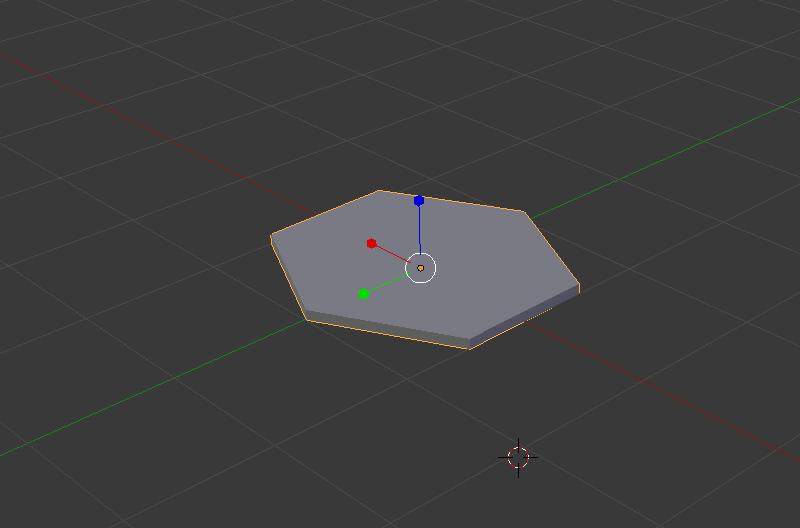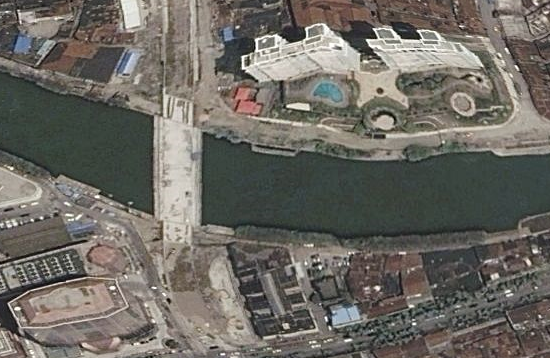## Posts Tagged ‘data visualization’

### Where we live

Using a number of technologies I’ve been playing around with recently, I began working on a 3D visualization of the Earth, plotting every city, creating a pointillism-styled representation of the planet. Below is the result along with an overview of how I produced the rendering.

#### Getting the data

I extracted all cities with a population of at least 100,000 people from the MySQL GeoNames database using the following query:

`SELECT `id`,`name`,`latitude`,`longitude`,`population`,`timezone`FROM geonames.citiesWHERE population >= 100000 AND feature_class = 'P';`

… and put the results into a JS array.

#### Creating a 3D model to represent each city

I created this hexagonal model in Blender, exported it to a Wavefront OBJ file, and ran the OBJ file through the Wavefront OBJ to JSON converter I wrote. Note that the model is facing the z-axis to match WebGL’s (and OpenGL’s) default camera orientation: facing down the negative z-axis.#### Convert longitude and latitude to a 3D position

Converting a geodetic longitude, latitude pair to a 3D position involves doing a LLA (Longitude Latitude Altitude) to ECEF (Earth-Centered, Earth-Fixed) transformation. The code below implements this transform, converting the longitude and latitude of every city pulled from the GeoNames database into a 3D coordinate where we can render the hexagonal representation of the city.

`function llarToWorld(lat, lon, alt, rad) {                lat = lat * (Math.PI/180.0);    lon = lon * (Math.PI/180.0);    var f = 0; //flattening    var ls = Math.atan( Math.pow((1.0 - f),2) * Math.tan(lat) ); // lambda    var x = rad * Math.cos(ls) * Math.cos(lon) + alt * Math.cos(lat) * Math.cos(lon)    var y = rad * Math.cos(ls) * Math.sin(lon) + alt * Math.cos(lat) * Math.sin(lon)    var z = rad * Math.sin(ls) + alt * Math.sin(lat)        return [x,z,-y];            }`

There are 2 items worth noting:

• The transformation (and function above) involve a 4th parameter, radius which is the radius of the ellipsoid (or sphere, in this case, as flattening=0) into which the transformation is done. I have it set as a fixed constant, as I’m primary concerned with an approximate visual representation, but the MathWorks page describes the actual computation.
• The ECEF (Earth-Centered, Earth-Fixed) coordinate system has the z-axis pointing north, not the y-axis, so the z and y values need to be swapped to produce a coordinate corresponding to WebGL’s default camera orientation. In addition, as WebGL has a right-handed coordinate system (so the default camera orientation is one where it’s pointing down the negative z-axis), the z coordinate is negated so the point doesn’t wind up behind the camera.

#### Orient all cities to face the origin

Getting each of the hexagonal models to face the origin involved a bit of math:

• Calculating the axis about which the rotation should occur by, first, computing a vector from the origin to the 3D position of the model (lookAt), and taking the cross product between lookAt and the z-axis (as we’re rotating toward the z-axis).
• Calculating the angle of rotation (the angle between the z-axis and lookAt) by computing the dot product between lookAt and the z-axis, then taking the acos of the dot product.

There’s some additional code to handle cases where points lie on the on the z-axis (where the cross product gives the zero vector) and also to return a matrix representation of the rotation.

` function lookAtOrigin(v) { // compute vector from origin var lookAt = vec3.create([v, v, -v]); vec3.normalize(lookAt); // reference axis var refAxis = vec3.create([0,0,-1]); // computate axis of rotation var rotAxis = vec3.create(lookAt); vec3.cross(rotAxis, refAxis); // compute angle of rotation var rotAngRad = Math.acos(vec3.dot(lookAt, refAxis)); // special cases... if(rotAxis == 0 && rotAxis == 0 && rotAxis == 0) { if(lookAt > 0) { rotAxis = vec3.create([1,0,0]); rotAngRad = Math.PI; } else { rotAxis = vec3.create([1,0,0]); rotAngRad = 0; } } // compute and return a matrix with the rotation var ret = mat4.identity(); mat4.rotate(ret, rotAngRad, rotAxis); return ret; }`

#### Render the scene

Using glfx, I pulled everything together, also adding a bit of code to rotate the camera and do some pseudo-lighting in the pixel shader by alpha blending colors based on depth. All the code can be found in the webgl-globe repository on bitbucket.

### Baidu Maps

Truly impressive: Baidu (“the Google of China”) doesn’t use satellite imagery for its maps, instead it uses isometric, Simcity-style sprites.It’s beautiful to look at, and with the crystal clear delineation of buildings, roadways, and landmarks, it’s likely a very effective navigational tool as well. The sheer scale of this blows my mind. I can’t even begin to fathom how much time and effort went into creating this much artwork to paint an entire city.

Bing’s bird eye view is the only service I can think of that comes close to this, but is far from impressive at times,### Spott map

Something pretty cool in MSSQL Server Management Studio: for columns with the geography data type, Management Studio will plot the points on a map. Here’s a map of all locations tagged from all dotspott users.### Wordle

Discovered wordle on someone’s twitter feed. I love this thing. Here’s the wordle of my post on Enyo:### The Great British Euler diagram

I finally understand…h/t to Things Of Interest, where you’ll also find a nice explanation to go along with the diagram.